Dr. J's Maths.com
Where the techniques of Maths
are explained in simple terms.

Trigonometric functions - Graphing and interpretation.
Test Yourself 1.

 Given an equation, describe the features (include references to shifts and dilations). 1. Describe the main features for the curve y = 3cos 2x. 2. Describe the main features for the curve y = 2 + sin x. 3. Describe the main features for the curve y = 2 - 3 cos 4x. 4. Describe the main features for the curve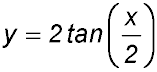. 5. Describe the main features for the curve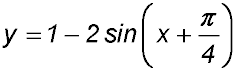Given a graph, interpret the features (include references to shifts and dilations). 6.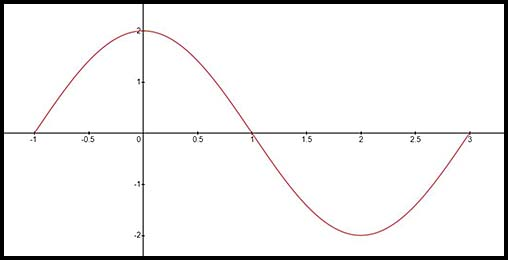The graph above can be represented by an equation of the form y = a cos nx. Find the values of a and n. Answer.a = 2 and n = π/2. 7. The equation of the following graph is written in the form y = A + B cos(Cx - D).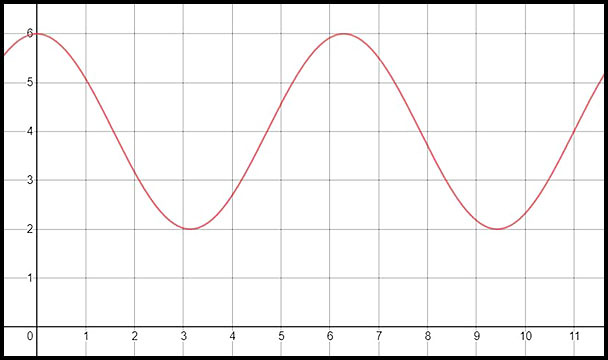Determine the values of A, B, C and D. Answer.A = 4, B = 2, C = 1 and D = 0. 8. The equation of the following graph is written in the form y = A + B tan(Cx + D).Determine the values of A, B, C and D. Answer.A = 0, B = 3, C = 2 and D = 0. 9. The equation of the following graph is written in the form y = A + B sin(Cx + D).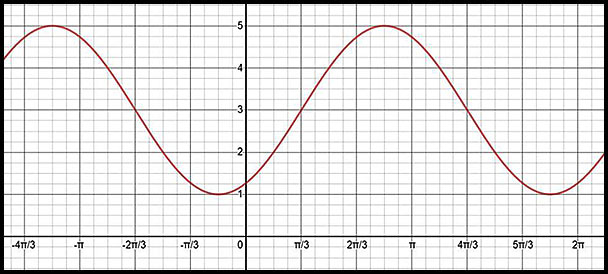Determine the values of A, B, C and D. Answer.A = 3, B = 2, C = 1 and D = -π/3. 10. The graph of D = A + Bcos Ct is given below.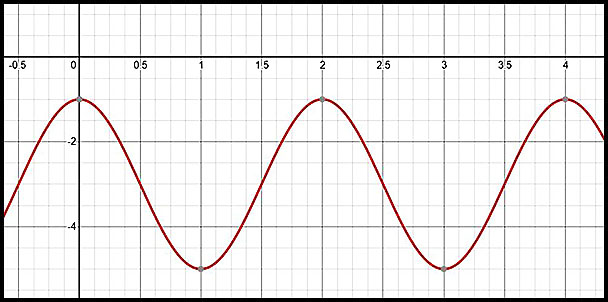(i) State the amplitude and period of the curve. (ii) Hence or otherwise determine the values of A, B and C. Answer.A = -3, B = 2, C = π. 11. What is the equation for the curve shown in the diagram below? Explain your reasons for your answer.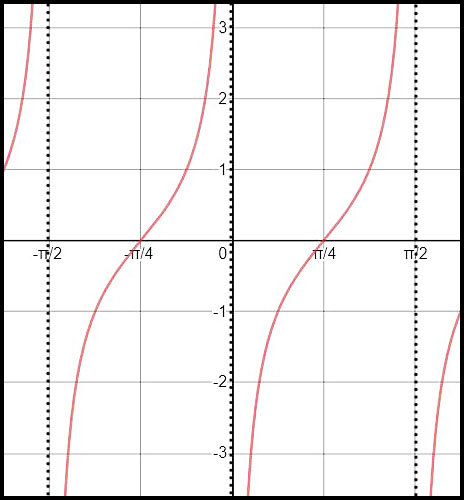Sketch graphs with transformations. 12. Sketch y = cos t + 2 for t:[0, 2π]. 13. For the function y = 2 cos x (i) State the amplitude of this function. (ii) Determine the period of the function. (iii) sketch the function for x:[0, 2π].. 14. For the function y = -2 sin 3x; (i) State the period. (ii) Sketch the graph of the function for x:[0, π]. 15. Draw sketches, on the same axes, of the curves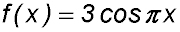and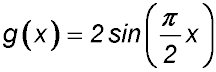in the domain of x:[0, 4]. 16. Sketch the curve y = 1 - 2 sin 2t for 0 ≤ t ≤ 2π. 17. Sketch the curve y = -3 + 2 cos πt for 0 ≤ t ≤ 2π. 18. Sketch the curve y = 2 + 3tan 2t for -π ≤ t ≤ π. 19. Sketch the curve y = 2 - 3 sin (t -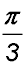) for 0 ≤ t ≤ 2π. 20. (i) Sketch the graph of y = 1 - 2cos x for 0 ≤x ≤ 2π. Clearly indicate the end points of the curve in its given domain as well as its turning points. (ii) Use your graph to solve 1 - 2cos x = 0 in the given domain. 21. (i) Sketch the curves y = sin x and y = cos x on the same axes for 0 ≤ x ≤ 2π. (ii) By adding ordinates, develop the graph of y = sin x + cos x. Graphs of reciprocal functions. 22. Sketch the curve y = 2 cosec 2x for 0° ≤ x ≤ 360°. 23. Sketch the curve y = cot 2t for 0° ≤ t ≤ 360°. 24. Sketch the curve y = sec x + 2 for 0 ≤x ≤ 2π. 25. Sketch the curve y = sec2 x for 0 ≤x ≤ 2π. 26. Sketch the curve y = 2 cot2 t for -π ≤ t ≤ π. 27. Sketch the curve y = 2 - cosec x for -3π/2 ≤ x ≤ 3π/2. Given features, draw the graph. 28. Draw a sine graph which has a maximum value of 7 and a minimum value of 1 and has two patterns in its domain of 0 ≤x ≤ 2π. 29. Draw a tan graph using the domain 0 ≤x ≤ 2π with the function having consecutive values of 0 at x = 0 and at x = π/4 and also a value of 5 at x = π/8. 30. Draw a sec graph which has minimum values of 2 at t = 0 and at t = 2π and a maximum value of -2 at t = π. Use the domain of -2π ≤ t ≤ 2π. 31. Draw a cosine graph having a minimum value of -4 at x = 0, a maximum value of 0 and having two patterns in its domain of 0 ≤x ≤ 2π. Determining the number of solutions. 32. (i) Sketch the graph of y = 2cos 2x for -π ≤ x ≤ π. (ii) On the same diagram, sketch the line x + y = 1. (iii) Hence determine the number of solutions of the equation 2 cos2x = 1 - x. (iv) Let the negative solution to 2 cos 2x = 1 - x be at x = N. Indicate N on the x-axis of your diagram. 33. (i) Sketch the graphs of y = 3 cos 2θ and y = 1 for 0 ≤ θ ≤ π. (ii) Use your graphs to obtain two approximate solutions to the equation. (iii) Solve the equation 3 cos 2θ = 1 using the normal technique for 0 ≤ θ ≤ π (answer to 3 decimal places) and compare your answers with those in part (ii). 34. (i) Sketch y = tan πx for 0 ≤ x ≤ 2. (ii) On the same diagram, sketch y = 1 - x. (iii) Hence determine the number of solutions to the equation tan πx = 1 - x for 0 ≤ x ≤ 2. 35. (i) Sketch the graph of y = sin 2x for 0 ≤ x ≤ π using relevant sub-intervals. (ii) Hence find the solutions to √2sin 2x > 1 for 0 ≤ x ≤ π. 36. (i) Draw a neat sketch of the curve y = 3sin 2x for 0 ≤ x ≤ 2π. (ii) On the same diagram, sketch y = 1 - cos x for 0 ≤ x ≤ 2π. (iii) Hence determine the number of solutions the equation 3 sin 2x + cos x = 1 will have in the given domain.